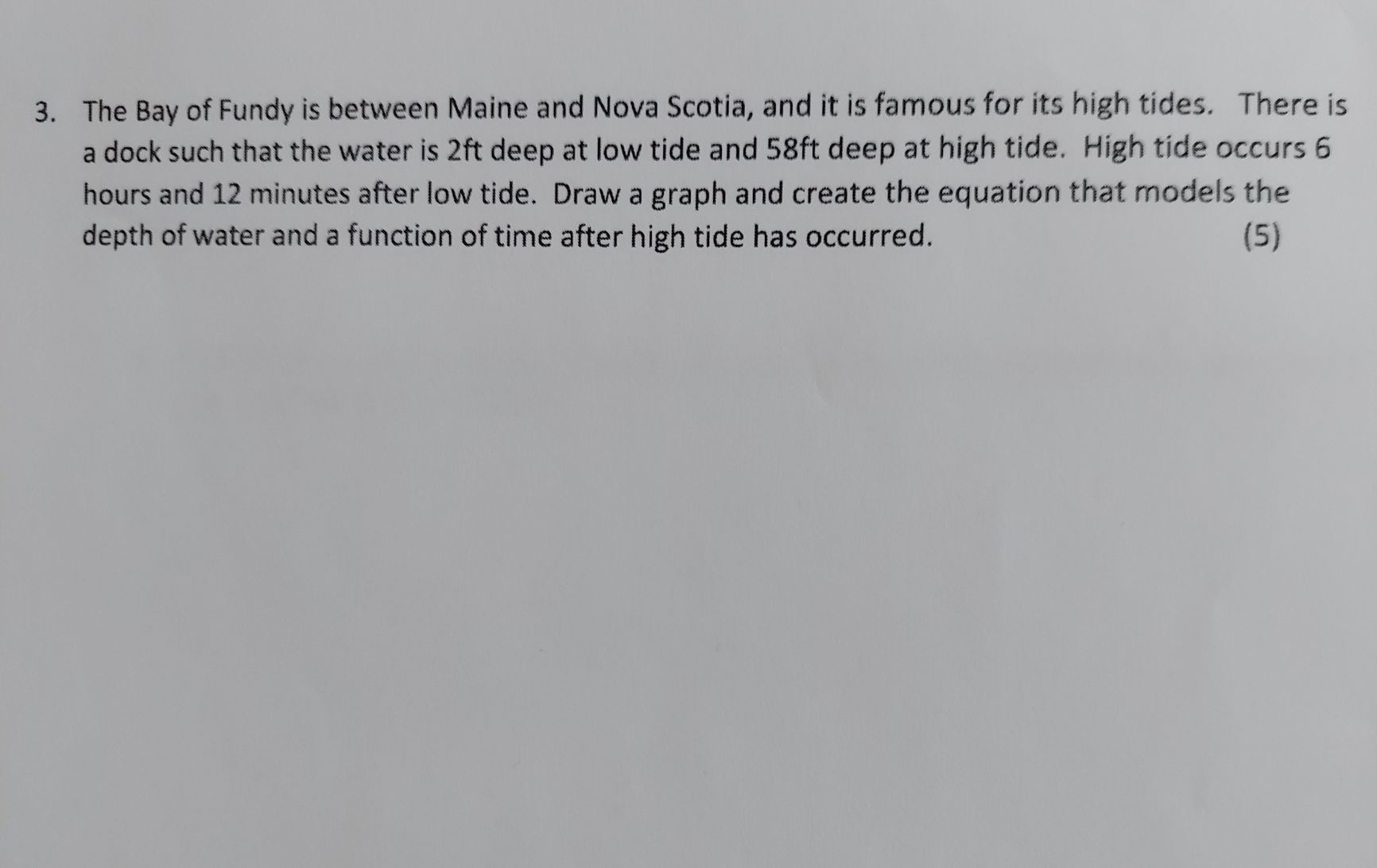### ¿Todavía tienes preguntas de matemáticas?

Pregunte a nuestros tutores expertos
Algebra
Pregunta3. The Bay of Fundy is between Maine and Nova Scotia, and it is famous for its high tides. There is a dock such that the water is $$2 ft$$ deep at low tide and $$58 ft$$ deep at high tide. High tide occurs $$6$$ hours and $$12$$ minutes after low tide. Draw a graph and create the equation that models the depth of water and a function of time after high tide has occurred.

$$h= \frac{280}{31}t + 2$$